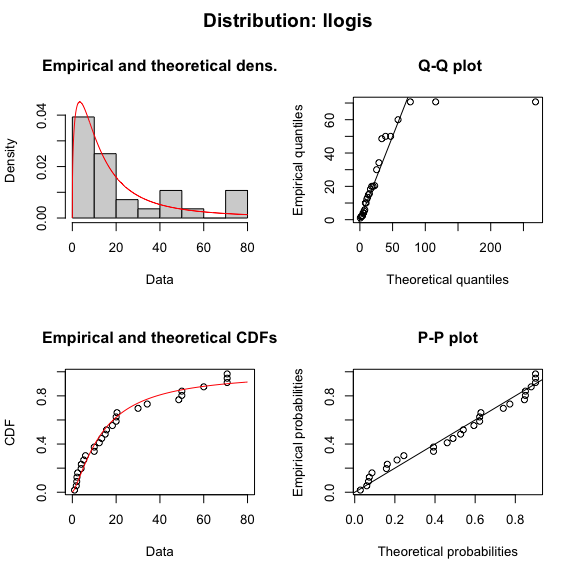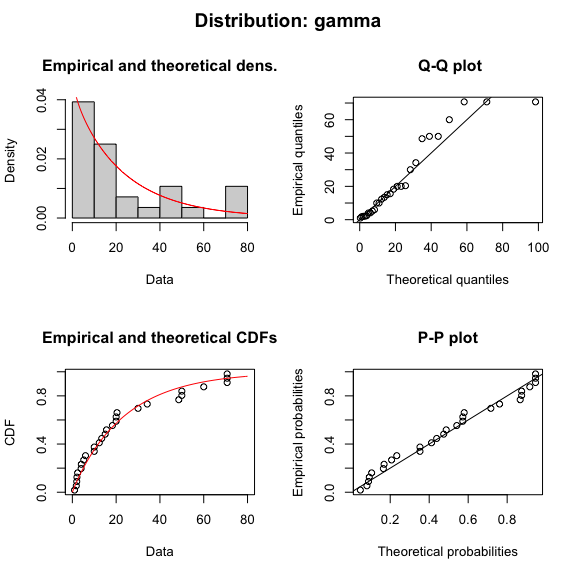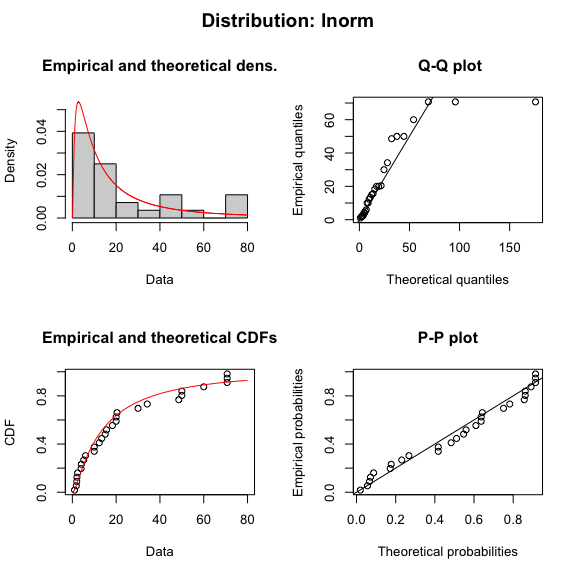#### 2021-10-27

library(ssdtools)
library(ggplot2)

## How do I fit a custom distribution?

The user can also specify a custom distribution (called say dist) provided the following functions are defined:

• probability density (ddist(x, par1, par2, log = FALSE))
• cumulative distribution (pdist(q, par1, par2, lower.tail = TRUE, log.p = FALSE))
• inverse cumulative distribution (qdist(p, par1, par2, lower.tail = TRUE, log.p = FALSE))
• random samples (rdist(n, par1, par2))
• starting values (sdist(x))

## How do I fit distributions to multiple groups such taxa and/or chemicals?

An elegant approach using some tidyverse packages is demonstrated below.

library(purrr)
library(tidyr)
library(dplyr)

boron_preds <- nest(ssdtools::boron_data, data = c(Chemical, Species, Conc, Units)) %>%
mutate(
Fit = map(data, ssd_fit_dists, dists = "lnorm"),
Prediction = map(Fit, predict)
) %>%
unnest(Prediction)

The resultant data and predictions can then be plotted as follows.

ssd_plot(boron_data, boron_preds, xlab = "Concentration (mg/L)", ci = FALSE) +
facet_wrap(~Group)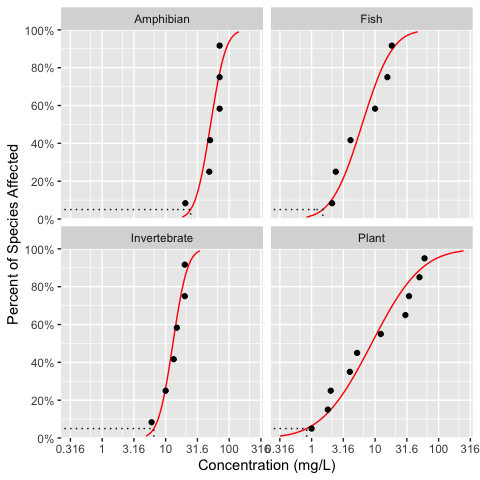### How do I plot a Cullen Frey plot?

The data can be visualized using a Cullen Frey plot of the skewness and kurtosis.

set.seed(10)
ssd_plot_cf(boron_data)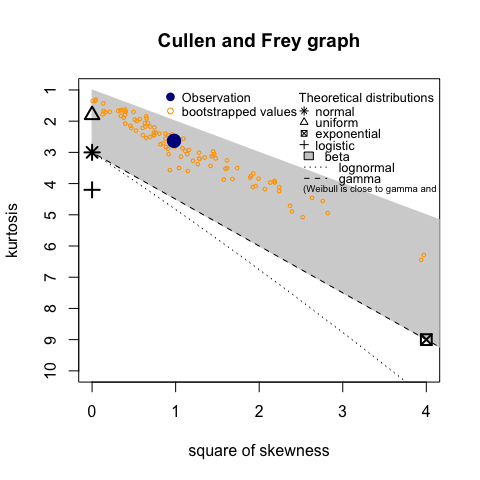## How do I plot model diagnostics?

A fitdists object can be plotted to display model diagnostics plots for each fit.

plot(boron_dists)